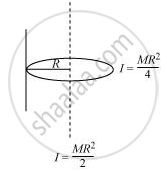# Given the Moment of Inertia of a Disc of Mass M and Radius R About Any of Its Diameters to Be Mr2/4, Find Its Moment of Inertia About an Axis Normal to the Disc and Passing Through a Point on Its Edge - Physics

#### Question

Given the moment of inertia of a disc of mass and radius about any of its diameters to be MR2/4, find its moment of inertia about an axis normal to the disc and passing through a point on its edge

#### Solution 1

3/2 MR^2

The moment of inertia of disc about its diameter  = 1/4 MR^2

According to the theorem of the perpendicular axis, the moment of inertia of a planar body (lamina) about an axis perpendicular to its plane is equal to the sum of its moments of inertia about two perpendicular axes concurrent with the perpendicular axis and lying in the plane of the body.

The M.I of the disc about its centre = 1/4 MR^2 + 1/4MR^2 = 1/2MR^2

The situation is shown in the given figureApplying the theorem of parallel axes:

The moment of inertia about an axis normal to the disc and passing through a point on its edge

= 1/2 MR^2 + MR^2 = 3/2 MR^2

#### Solution 2

We are given, moment of inertia of the disc about any of its diameters = 1/4 MR2

(i) Using theorem of perpendicular axes, moment of inertia of the disc about an axis passing through its centre and normal to the disc = 2 x 1/4 MR2 = 1/2 MR2

(ii) Using theorem axes, moment of inertia of the disc passing through a point on its edge and normal to the dies = 1/2 MR2+ MR2 = 3/2 MR2.

Is there an error in this question or solution?

#### APPEARS IN

NCERT Solution for Physics Textbook for Class 11 (2018 (Latest))
Chapter 7: System of Particles and Rotational Motion
Q: 10.1 | Page no. 178

#### Video TutorialsVIEW ALL 

Given the Moment of Inertia of a Disc of Mass M and Radius R About Any of Its Diameters to Be Mr2/4, Find Its Moment of Inertia About an Axis Normal to the Disc and Passing Through a Point on Its Edge Concept: Moment of Inertia.